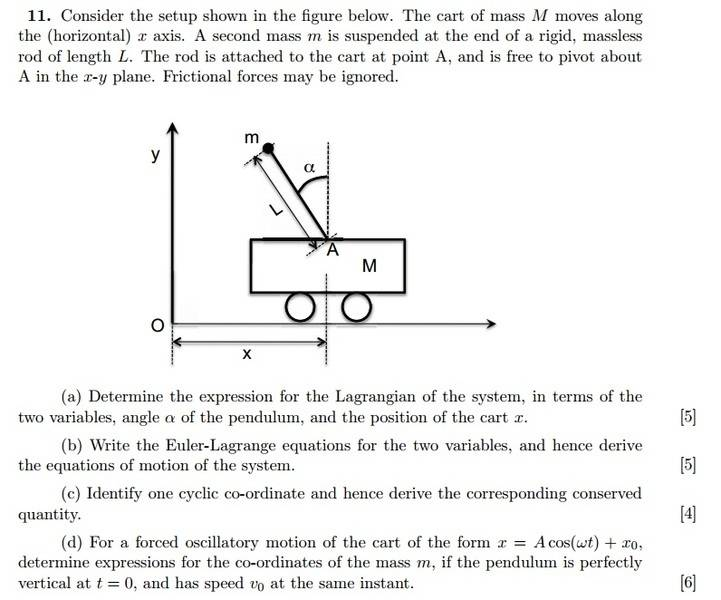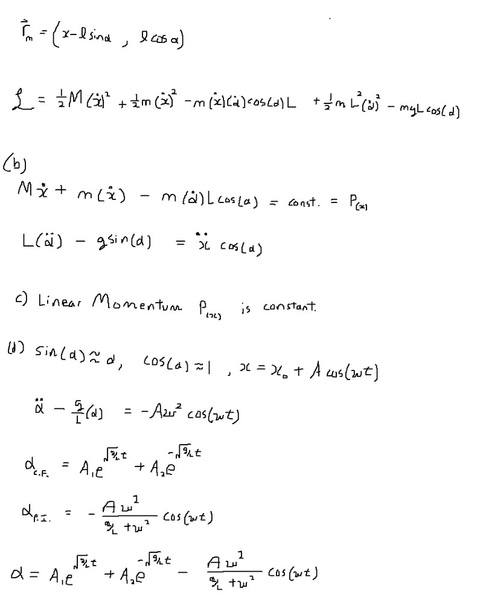# Lagrangian question, inverted pendulum (Very near to the answer!)

## Homework Statement

Consider the setup shown in the gure below. The cart of mass M moves along
the (horizontal) x axis. A second mass m is suspended at the end of a rigid, massless
rod of length L. The rod is attached to the cart at point A, and is free to pivot about A in the x-y plane. Frictional forces may be ignored.## Homework Equations

I got the same lagrangian as in wikipedia, so i think it's right.
http://en.wikipedia.org/wiki/Inverted_pendulum

## The Attempt at a Solution## Answers and Replies

Your Lagrangian and equations of motions are correct. I'm pretty sure A1 has to be zero so that your solution does not blow up at infinity. Then you can use the initial conditions given in the problem to solve for A2 and A

Your Lagrangian and equations of motions are correct. I'm pretty sure A1 has to be zero so that your solution does not blow up at infinity. Then you can use the initial conditions given in the problem to solve for A2 and A

I know right? But I can't find any mathematical reason why A1 = 0...I get 2 values of β in the characteristic equation where α = A*exp(βt)..